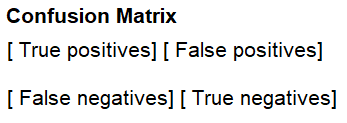﻿ How to Create a Confusion Matrix in Python using sklearn ﻿# How to Create a Confusion Matrix in Python using sklearnIn this article, we show how to create a confusion matrix in Python using the sklearn module.

A confusion matrix is a matrix that tells us what a machine learning program got correct and what it got wrong.

The confusion matrix gives us these results in terms of true positives, false positives, true negatives and false negatives.

The confusion matrix returns a matrix of 4 results in integer values.

A diagram that illustrates the confusion matrix is shown below.So the first top left value is true positives. These are the results that the machine learning program classifies correctly as positive results.

The right top value is false positives. These are the results that the machine learning program classifies wrongly as positive results (when they are actually negative results).

The bottom left value is false negatives. These are results that the machine learning program classifies wrongly as negative results (when they are actually positive results).

The bottom right value is positive negatives. These are results that the machine learning program classifies correctly as negative results.

So the sklearn module in Python uses this convention when giving us a confusion matrix.

It helps us to know how well our machine learning program is functioning with test data, how accurate it is.

If the program is giving us a lot of errors, false positives or false negatives, then we know the machine learning program is either inappropriate or needs more training data to function well.

So below we have a decision tree classifier that classifies outcomes based on given data. It represents whether children will go outside to play (positive result) or not (negative results) based on a few weather variables (temperature, humidity, and whether it is windy).

The CSV file used with this machine learning program can be found at the following link: Play.csv

As an output, we create a confusion matrix which represents a metric that allows us to see how well the program performed.

So in order to create a confusion matrix, we have to import confusion_matrix from sklearn.metrics.

A confusion matrix is a metric that allows us to see if our machine learning program is effective or not, and it does this through telling us the number of true positives, false positives, false negatives, and true negatives.

The results of our program is shown below.

So we can see in the above results that the test data set had 7 data points or examples fed into the machine learning program.

The machine learning program classified 3 results as true positives and 4 results as true negatives. There were no false positives or false negatives. Thus, our program performed with 100% efficiency, which is good.

You may think that this 7 data points is too few. If you want to give the program more test points, you can either create a larger training data set or increase the test_size value fed into the train_test_split() function. This will give more data points so that we can get an idea of how our machine learning program works with a larger data set.

And this is how to create a confusion matrix in Python using the sklearn module.

Related Resources

How to Randomly Select From or Shuffle a List in Python